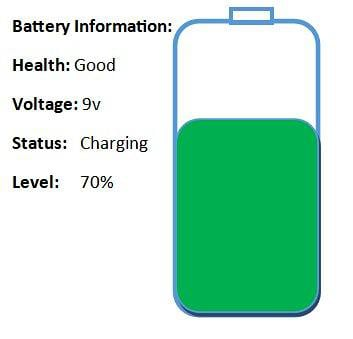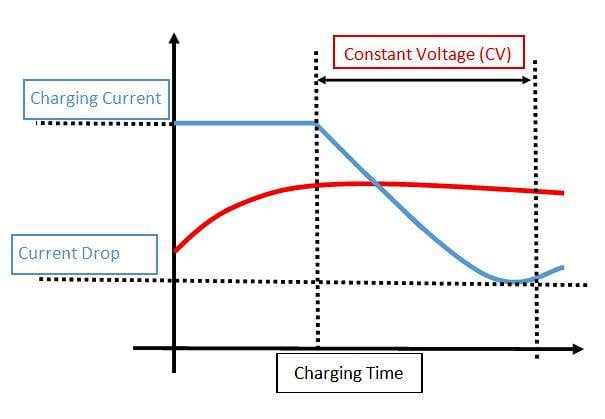Technical Article

# Evaluating Battery Charger Voltage Characteristics

February 03, 2023 by Bob Odhiambo

## This article focuses on the voltage characteristics in battery chargers and their relation to battery charging and examines voltage charging methods.

A battery charger restores charge to a battery by allowing the flow of electric current. The protocol in which the charging takes place is dependent on factors such as voltage, current, and battery size. This technical article will look into voltage characteristics and their relation to battery charging.

### Voltage Overview

Voltage is the electrical potential difference (p.d) between two points in a circuit. This potential difference is a result of charge build-up between the two points.

The SI voltage unit is Volts denoted by (V) and is derived from other quantities as indicated in the expression below

Voltage = Energy ÷ Charge

### State of Charge and Depth of Discharge

State of charge (SOC) is the charge level in a battery to its capacity. The level of SOC is shown by a percentage where 0% indicates empty, and 100% indicates the battery capacity is full.

Depth of discharge (DOD) is the reverse of SOC as it indicates the percentage of voltage drained from the battery. For example, if a battery is at 70% LEVEL, its DOD value is 30% because it is the amount drained off the battery.### Understanding Voltage Characteristics

Understanding voltage characteristics is vital for the following reasons

• Safety. For safe battery charging, a better understanding of voltage characteristics is required. This understanding ensures the battery does not explode due to an overcharge or get damaged due to an undercharge.
• Battery life. For longer battery life, it is crucial to understand its voltage characteristics because an overcharge or an undercharge of the battery can shorten its lifespan.
• Efficiency. With the knowledge of voltage characteristics, the efficiency of a battery charging system can be optimized and thus can be charged efficiently and fast.
• Cost Effectiveness. The cost incurred from battery replacement can be reduced by ensuring the battery is charged properly.
• Reliability. Properly charging a battery makes it more reliable than a poorly charged battery, which can cause operational downtime.

### Voltage Charging Methods

There are several types of voltage charging methods, including the following:

#### Constant Voltage (CV) Charging

The constant voltage method keeps a constant voltage during the charging process. However, there is a gradual decrease in current as the battery charges. The charging process stops after this current reaches a certain level. This charging method is used in nickel-cadmium and lead-acid batteries.#### Pulse Charging

In pulse charging, bursts of current rather than continuous current charge the battery. The pulse generation uses a specialized charging algorithm. The main advantage of this charging method is that it can prolong the battery's lifespan. The method also helps prevent the build-up of lead sulfate that forms on the surface of the battery plate over time.

#### Temperature-compensated Charging

In this method, the charging voltage is either decreased or increased due to changes in battery temperature. The adjustments are made to compensate for the effects of temperature on battery charging characteristics.

### Factors Affecting Battery Charging Voltage

Several factors affect the voltage in battery charging, including:

#### Battery Capacity

Battery capacity is the amount of current in a battery and is expressed in ampere-hours (Ah). This energy stored in a battery can also be expressed as watt-hours (Wh) and kilowatt-hours (KWh). A more accurate voltage variation can be accounted for by multiplying Ah by the battery voltage and the charging time. This will result in the watt-hours value of the battery. Batteries with larger battery capacity will require high charging voltage.

#### Battery Type

Battery type affects voltage in charging because of the varying charging characteristics in different batteries. For instance, lead-acid batteries need a charging voltage of approximately 14.4 V, whereas lithium-ion batteries require 4.2 V for each cell. The charging method can also vary in different battery types.

#### Battery Age

As the battery ages, it loses its ability to retain a charge. This means that the voltage level retained by the battery will drop and, when charged, will not reach the same level as a brand-new battery. Due to this fact, the lifespan of the battery will be short.

#### Temperature

An increase in temperature affects the battery by decreasing its internal resistance. With the resistance being less, more current can flow into the battery. This implies that the charging voltage needed for the battery will be higher. This effect, where temperature affects the charging voltage of the battery, is called the thermoelectric effect.

#### Battery Impedance

Battery impedance is the resistance within the battery that opposes the current flow. High impedance can lead to slow charging because the higher impedance will impede the current flow, resulting in a lower voltage across the terminals.

#### Charger Specifications

The specifications of battery chargers may vary with different battery types. They often specify the voltage and current output that can affect the charging process. A charger with low output voltage may not be able to charge a battery to its full capacity. On the other hand, a higher voltage output could damage the battery or shorten its lifespan.

### Voltage Measurement Methods

The following are methods used to measure voltage.

#### Direct Voltage Measurement

In this method, the voltage reading is acquired directly from the circuit with measuring equipment such as a multimeter. A multimeter is electronic equipment that can measure a variety of electrical quantities, such as voltage, resistance, and current.

Another piece of equipment that can acquire voltage data directly is the Oscilloscope which visually displays electric signals on a screen in waves.

This measuring equipment has probes placed directly on the voltage source to acquire the measurements.

#### Indirect Voltage Measurement

In this method, voltage reading is acquired in a simple calculation using Ohm's Law (V= I x R), where current (I) and resistance (R) are known.

Below are some methods to acquire voltage:

Voltage divider circuit: This circuit divides the voltage across a series of resistors whose readings can be acquired using a multimeter.

Thevenin's circuit theorem: In this method, the voltage across specific components in a circuit can be acquired by replacing the other component with their internal resistance.

Ohm's law: By measuring the current and resistance across a circuit, the voltage can be derived from Ohm's law equation (V= I x R).

#### RMS Voltage Measurement

This technique measures the effective value of an alternating current (AC) waveform. This waveform, also known as a steady state voltage, produces similar heat dissipation in a resistive load as in an actual AC waveform.

Use the formula below to calculate the root mean square (RMS):

$Vrms=\sqrt{\frac{1}{T}}\int\limits_{to}^{t0+T}v^{2}(t)dt$

Where T is the period of waveform and V(t) is the instantaneous voltage at time t.

RMS readings can be acquired by a specialized voltmeter known as an RMS voltmeter. This measuring device converts the AC waveform to DC waveform for higher precision and accuracy.

### Key Takeaways of Battery Charging Voltage

Following are some key takeaways of voltage characteristics in battery chargers.

• Understanding voltage characteristics is essential for the safety, life, efficiency of a battery, and reliability of the equipment it powers.
• For voltage charging, constant voltage, pulse method, and temperature-compensation charging methods can be used.
• Factors affecting battery charging include battery capacity, type, age, temperature, impedance, and charger specification.
• A multimeter and an oscilloscope are instruments used to get voltage readings.
• Voltage reading can be acquired using Ohm's law equation V = I x R.
• The formula below can be used to calculate the root mean square:

$Vrms=\sqrt{\frac{1}{T}}\int\limits_{to}^{t0+T}v^{2}(t)dt$

Featured image used courtesy of Adobe Stock